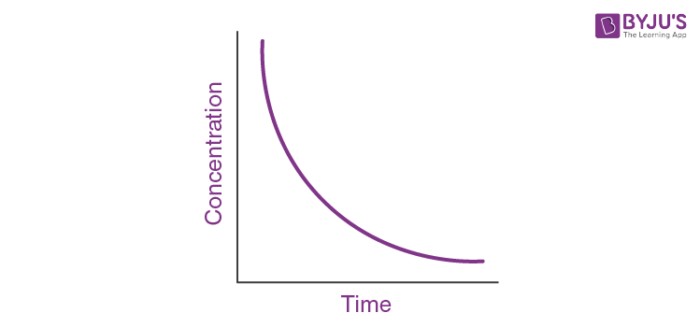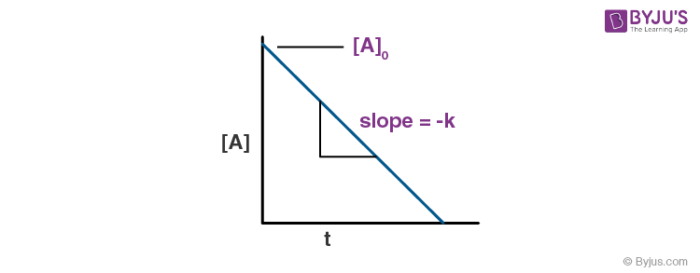# First-Order Reactions

## Introduction

A first-order reaction is one in which the rate of reaction is proportional to the concentration of the reactant. To put it another way, doubling the concentration doubles the reaction rate. A first-order reaction can have one or two reactants, as in the case of the decomposition reaction.

## What is a First-Order Reaction?

A first-order reaction can be defined as a chemical reaction in which the reaction rate is linearly dependent on the concentration of only one reactant. In other words, a first-order reaction is a chemical reaction in which the rate varies based on the changes in the concentration of only one of the reactants. Thus, the order of these reactions is equal to 1.

### Examples of First-Order Reactions

• SO2Cl2 → Cl2 + SO2
• 2N2O5 → O2 + 4NO2
• 2H2O2 → 2H2O + O2

## Differential Rate Law for a First-Order Reaction

A differential rate law can be employed to describe a chemical reaction at a molecular level. The differential rate expression for a first-order reaction can be written as:

Rate = -d[A]/dt = k[A]1 = k[A]

Where,

• ‘k’ is the rate constant of the first-order reaction, whose units are s-1.
• ‘[A]’ denotes the concentration of the first-order reactant ‘A’.
• d[A]/dt denotes the change in the concentration of the first-order reactant ‘A’ in the time interval ‘dt’.

## Integrated Rate Law for a First-Order Reaction

Integrated rate expressions can be used to experimentally calculate the value of the rate constant of a reaction. To obtain the integral form of the rate expression for a first-order reaction, the differential rate law for the first-order reaction must be rearranged as follows.

$$\begin{array}{l}\frac{-d[A]}{dt} = k[A]\end{array}$$
$$\begin{array}{l}\Rightarrow \frac{d[A]}{[A]} = -kdt\end{array}$$

Integrating both sides of the equation, the following expression is obtained.

$$\begin{array}{l}\int_{[A]_0}^{[A]}\frac{d[A]}{[A]} = -\int_{t_0}^{t}kdt\end{array}$$

Which can be rewritten as:

$$\begin{array}{l}\int_{[A]_0}^{[A]}\frac{1}{[A]}d[A] = -\int_{t_0}^{t}kdt\end{array}$$

Since

$$\begin{array}{l}\int\frac{1}{x} = ln(x) \end{array}$$
, the equation can be rewritten as follows:

ln[A] – ln[A]0 = -kt

ln[A] = -kt + ln[A]0 (or) ln[A] = ln[A]0 – kt

Raising each side of the equation to the exponent ‘e’ (since eln(x) = x), the equation is transformed as follows:

$$\begin{array}{l}e^{ln[A]} = e^{ln[A]_0 – kt}\end{array}$$

Therefore,

$$\begin{array}{l}[A] = [A]_0 e^{-kt}\end{array}$$

This expression is the integrated form of the first-order rate law.

## Graphical Representation of a First-Order Reaction

The concentration v/s time graph for a first-order reaction is provided below.For first-order reactions, the equation ln[A] = -kt + ln[A]0 is similar to that of a straight line (y = mx + c) with slope -k. This line can be graphically plotted as follows.Thus, the graph for ln[A] v/s t for a first-order reaction is a straight line with slope -k.

## Half-Life of a First-Order Reaction

The half-life of a chemical reaction (denoted by ‘t1/2’) is the time taken for the initial concentration of the reactant(s) to reach half of its original value. Therefore,

At t = t1/2 , [A] = [A]0/2

Where [A] denotes the concentration of the reactant and [A]0 denotes the initial concentration of the reactant.

Substituting the value of A = [A]0/2 and t = t1/2 in the equation [A] = [A]0 e-kt:

$$\begin{array}{l}\frac{[A]_0}{2} = [A]_0 e^{-kt_{1/2}}\end{array}$$
$$\begin{array}{l}\Rightarrow \frac{1}{2} = e^{-kt_{1/2}}\end{array}$$

Taking the natural logarithm of both sides of the equation to eliminate ‘e’, the following equation is obtained.

$$\begin{array}{l}ln(\frac{1}{2}) = -kt_{1/2}\end{array}$$
$$\begin{array}{l}\Rightarrow t_{1/2} = \frac{0.693}{k}\end{array}$$

Thus, the half-life of a first-order reaction is equal to 0.693/k (where ‘k’ denotes the rate constant, whose units are s-1).

## Frequently Asked Questions – FAQs

Q1

### What is the definition of a first-order reaction?

A first-order reaction can be defined as a chemical reaction for which the reaction rate is entirely dependent on the concentration of only one reactant. In such reactions, if the concentration of the first-order reactant is doubled, then the reaction rate is also doubled. Similarly, if the first-order reactant concentration is increased five-fold, it will be accompanied by a 500% increase in the reaction rate.

Q2

### What are the differential rate law and the integrated rate law for a first-order reaction?

The differential rate law for a first-order reaction can be expressed as follows:

Rate = -d[A]/dt = k[A]

The integrated rate equation for a first-order reaction is:

[A] = [A]0e-kt

Where,

• [A] is the current concentration of the first-order reactant
• [A]0 is the initial concentration of the first-order reactant
• t is the time elapsed since the reaction began
• k is the rate constant of the first-order reaction
• e is Euler’s number (which is the base of the natural logarithm)
Q3

### What is the relationship between the half-life and the rate constant for a first-order reaction?

The half-life of a chemical reaction is the time required for the concentration of the reactants to reach half of their initial value. For first-order reactions, the relationship between the reaction half-life and the reaction rate constant is given by the expression:

t1/2 = 0.693/k

Where ‘t1/2’ denotes the half-life of the reaction and ‘k’ denotes the rate constant.

Q4

### What are the units of the rate constant for a first-order reaction?

For first-order reactions, the rate constant is expressed in s1 (reciprocal seconds). The units of the rate constant can be determined using the following expression:

Units of k = M(1-n).s-1 (where ‘n’ is the order of the reaction)

Since the reaction order of a first-order reaction is equal to 1, the equation is transformed as follows:

Units of k = M(1-1).s-1 = s-1

Q5

### For a first-order reaction, if a graph is plotted with ln[A] on the Y-axis and time on the X-axis, what will it look like?

The graph will be a straight line with a slope of -k.